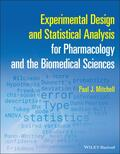John Wiley & Sons Experimental Design and Statistical Analysis for Pharmacology and the Biomedical SciencesExperimental Design and Statistical Analysis for Pharmacology and the Biomedical Sciences A practic.. Product #: 978-1-119-43763-5 Regular price: \$40.09 \$40.09 Auf Lager

# Experimental Design and Statistical Analysis for Pharmacology and the Biomedical Sciences1. Auflage Mai 2022
256 Seiten, Softcover
Wiley & Sons Ltd

ISBN: 978-1-119-43763-5
John Wiley & Sons

Jetzt kaufen

### Preis: 42,90 €

Preis inkl. MwSt, zzgl. Versand

Experimental Design and Statistical Analysis for Pharmacology and the Biomedical Sciences

A practical guide to the use of basic principles of experimental design and statistical analysis in pharmacology

Experimental Design and Statistical Analysis for Pharmacology and the Biomedical Sciences provides clear instructions on applying statistical analysis techniques to pharmacological data. Written by an experimental pharmacologist with decades of experience teaching statistics and designing preclinical experiments, this reader-friendly volume explains the variety of statistical tests that researchers require to analyze data and draw correct conclusions.

Detailed, yet accessible, chapters explain how to determine the appropriate statistical tool for a particular type of data, run the statistical test, and analyze and interpret the results. By first introducing basic principles of experimental design and statistical analysis, the author then guides readers through descriptive and inferential statistics, analysis of variance, correlation and regression analysis, general linear modelling, and more. Lastly, throughout the textbook are numerous examples from molecular, cellular, in vitro, and in vivo pharmacology which highlight the importance of rigorous statistical analysis in real-world pharmacological and biomedical research.

This textbook also:
* Describes the rigorous statistical approach needed for publication in scientific journals
* Covers a wide range of statistical concepts and methods, such as standard normal distribution, data confidence intervals, and post hoc and a priori analysis
* Discusses practical aspects of data collection, identification, and presentation
* Features images of the output from common statistical packages, including GraphPad Prism, Invivo Stat, MiniTab and SPSS

Experimental Design and Statistical Analysis for Pharmacology and the Biomedical Sciences is an invaluable reference and guide for undergraduate and graduate students, post-doctoral researchers, and lecturers in pharmacology and allied subjects in the life sciences.

Foreward 4

1 Introduction 6

2 So, what are data? 8

3 Numbers; counting and measuring, precision and accuracy 9

4 Data collection: Sampling and populations, different types of data, data distributions 12

5 Descriptive statistics: measures to describe and summarize data sets. 16

6 Testing for Normality and transforming skewed data sets 22

7 The Standard Normal Distribution 28

8 Non-Parametric Descriptive statistics 30

9 Summary of descriptive statistics; so, what values may I use to describe my data? 34

Decision Flowchart 1: Descriptive Statistics - Parametric v Non-parametric data 43

10 Introduction to Inferential statistics 44

11 Comparing 2 sets of data - Independent t-test 50

12 Comparing 2 sets of data - Paired t-test 55

13 Comparing 2 sets of data - Independent non-parametric data 58

14 Comparing 2 sets of data - Paired non-parametric data 62

15 Parametric 1-way Analysis of Variance 66

16 Repeated Measures Analysis of Variance 78

17 Complex Analysis of Variance models 86

18 Non-parametric ANOVA 102

Decision Flowchart 2: Inferential Statistics - Single and multiple pairwise comparisons 115

19 Correlation Analysis 116

20 Regression Analysis 126

21 Chi-Square Analysis 136

Decision Flowchart 3: Inferential Statistics -Tests of Association 145

22 Confidence Intervals 146

23 Permutation Test of Exact Inference 150

24 General Linear Model 152

Appendices Introduction to Appendices 155

A Data distribution: probability mass function and probability density functions

A.1 Binomial Distribution 156

A.2 Exponential Distribution 157

A.3 Normal Distribution 158

A.4 Chi-square Distribution 159

A.5 Student t-Distribution 160

A.6 F Distribution 161

B Standard Normal Probabilities

B.1 AUC values for z values below the mean (i.e. -z) 162

B.2 AUC values for z values above the mean (i.e. +z) 163

C Critical values of the t-distribution 164

D Critical values of the Mann-Whitney U statistic

D.1 Critical values for U; One-tailed test, p = 0.05 165

D.2 Critical values for U; One-tailed test, p = 0.01 166

D.3 Critical values for U; Two-tailed test, p = 0.05 167

D.4 Critical values for U; Two-tailed test, p = 0.01 168

E Critical values of the F distribution

E.1 Critical values of F, p = 0.05 169

E.2 Critical values of F, p = 0.01 170

E.3 Critical values of F, p = 0.001 171

F Critical values of the Chi-square distribution 172

G Critical z values for multiple non-parametric pairwise comparisons

G.1 Critical values of z according to the number of comparisons 173

G.2 Alternative critical values of z according to the number of comparisons when all groups have an equal number of subjects 173

H Critical values of correlation coefficients

H.1 Pearson Product Moment Correlation 174

H.2 Spearman Rank Correlation 174

H.3 Kendall's Rank Correlation (Kendall's tau) 175

Overall Decision Flowchart: Descriptive and Inferential Statistics 176

Index
Dr Paul J. Mitchell is Senior Lecturer and Associate Professor in the Department of Pharmacy and Pharmacology, University of Bath, UK, and Adjunct Lecturer in the Department of Pharmacology and Therapeutics, National University of Ireland (NUI), Galway, Ireland. He has more than 45 years' experience in experimental pharmacology, experimental design, and statistical analysis. For the last 25 years Dr Mitchell has collaborated with colleagues to develop a coherent strategy to teach experimental design and statistical analysis to undergraduate and graduate students across subject areas.